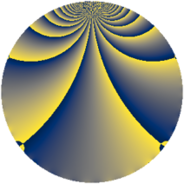# Properties

 Label 363.3.oLevel $363$ Weight $3$ Character orbit 363.o Rep. character $\chi_{363}(7,\cdot)$ Character field $\Q(\zeta_{110})$ Dimension $1760$ Newform subspaces $1$ Sturm bound $132$ Trace bound $0$

# Related objects

## Defining parameters

 Level: $$N$$ $$=$$ $$363 = 3 \cdot 11^{2}$$ Weight: $$k$$ $$=$$ $$3$$ Character orbit: $$[\chi]$$ $$=$$ 363.o (of order $$110$$ and degree $$40$$) Character conductor: $$\operatorname{cond}(\chi)$$ $$=$$ $$121$$ Character field: $$\Q(\zeta_{110})$$ Newform subspaces: $$1$$ Sturm bound: $$132$$ Trace bound: $$0$$

## Dimensions

The following table gives the dimensions of various subspaces of $$M_{3}(363, [\chi])$$.

Total New Old
Modular forms 3600 1760 1840
Cusp forms 3440 1760 1680
Eisenstein series 160 0 160

## Trace form

 $$1760 q - 100 q^{4} + 4 q^{5} + 30 q^{7} + 40 q^{8} - 1320 q^{9} + O(q^{10})$$ $$1760 q - 100 q^{4} + 4 q^{5} + 30 q^{7} + 40 q^{8} - 1320 q^{9} + 88 q^{10} - 100 q^{11} + 24 q^{12} + 58 q^{13} - 78 q^{14} + 24 q^{15} + 168 q^{16} + 10 q^{17} + 30 q^{18} - 2 q^{20} + 46 q^{22} + 252 q^{23} - 90 q^{24} + 202 q^{25} - 86 q^{26} + 50 q^{28} - 160 q^{29} - 180 q^{30} - 12 q^{31} - 12 q^{33} + 112 q^{34} + 320 q^{35} - 300 q^{36} + 340 q^{37} + 98 q^{38} - 30 q^{40} + 120 q^{41} + 180 q^{42} + 492 q^{44} + 12 q^{45} - 50 q^{46} + 114 q^{47} + 96 q^{48} - 554 q^{49} + 1958 q^{50} + 60 q^{51} - 396 q^{52} - 58 q^{53} - 596 q^{55} - 1058 q^{56} - 60 q^{57} - 504 q^{58} - 74 q^{59} + 90 q^{61} + 1170 q^{62} - 90 q^{63} - 100 q^{64} - 462 q^{65} - 48 q^{66} - 50 q^{67} - 80 q^{68} - 162 q^{69} + 344 q^{70} + 1044 q^{71} + 150 q^{72} + 636 q^{73} + 730 q^{74} + 360 q^{75} + 3234 q^{76} + 390 q^{77} - 450 q^{78} - 782 q^{79} + 666 q^{80} - 3960 q^{81} + 378 q^{82} + 190 q^{83} + 180 q^{84} + 682 q^{85} - 708 q^{86} + 648 q^{88} + 932 q^{89} + 204 q^{90} + 408 q^{91} - 1702 q^{92} - 120 q^{93} + 350 q^{94} - 430 q^{95} - 1242 q^{96} - 208 q^{97} + 1232 q^{98} - 510 q^{99} + O(q^{100})$$

## Decomposition of $$S_{3}^{\mathrm{new}}(363, [\chi])$$ into newform subspaces

Label Dim $A$ Field CM Traces $q$-expansion
$a_{2}$ $a_{3}$ $a_{5}$ $a_{7}$
363.3.o.a $1760$ $9.891$ None $$0$$ $$0$$ $$4$$ $$30$$

## Decomposition of $$S_{3}^{\mathrm{old}}(363, [\chi])$$ into lower level spaces

$$S_{3}^{\mathrm{old}}(363, [\chi]) \cong$$ $$S_{3}^{\mathrm{new}}(121, [\chi])$$$$^{\oplus 2}$$